# No, not there...

Tags

- - CUT SCREEN HERE - -

Write a program telling how many peanuts to get, depending on an animal type. You can try it for a goat: https://webappexamples.skilling.us/html/no-not-there/no-not-there.php?animal=goat

Here's some sample output, for `?animal=goat` at the end of the URL: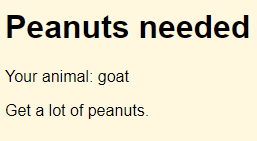All input is in lowercase.

Here are the messages for different animals:

Animal Message
Squirrel Get a few peanuts.
Goat Get a lot of peanuts.
Elephant Get lots and lots and lots of peanuts!
Other I don't know how many peanuts you'll need.

- - CUT SCREEN HERE - -

Let's watch Ray work through it.Ray

OK, looks easy enough. I'll start by checking the example in the last lesson.

GET... `if`, `==`... OK.

So, start by GETting input. The field is called animal, so...

• <?php
• // Get input.
• \$animal = \$_GET['animal'];

Hmm... the output wants to show the animal.Looks like a `p` tag. Let me check. I'll look at the sample page, then check the HTML on it: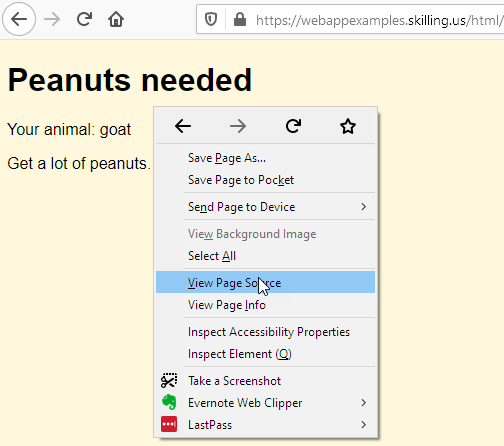That will show me the HTML created by the program.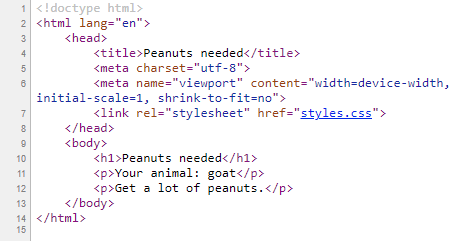I'll find the HTML showing the animal: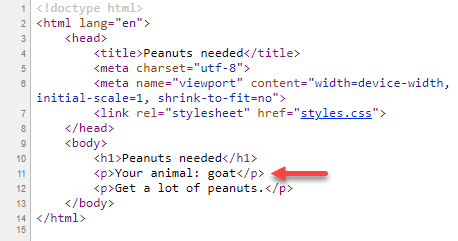Right, it's a `p` tag. So...

• <?php
• // Get input.
• \$animal = \$_GET['animal'];
• print "<p>Your animal: \$animal </p>";

Is that right?

Is the code right so far?Ray

Now for the `if`. Start with squirrels. Let me check the output message:

Animal Message
Squirrel Get a few peanuts.
Goat Get a lot of peanuts.
Elephant Get lots and lots and lots of peanuts!
Other I don't know how many peanuts you'll need.

So that would be...

1. <?php
2. // Get input.
3. \$animal = \$_GET['animal'];
4. print "<p>Your animal: \$animal </p>";
5. // Print right message.
6. if (\$animal == 'squirrel') {
7.     print '<p>Get a few peanuts.</p>';
8. }

OK, now the others...

1. <?php
2. // Get input.
3. \$animal = \$_GET['animal'];
4. print "<p>Your animal: \$animal </p>";
5. // Print right message.
6. if (\$animal == 'squirrel') {
7.     print '<p>Get a few peanuts.</p>';
8. }
9. elseif (\$animal == 'goat') {
10.     print '<p>Get a lot of peanuts.</p>';
11. }
12. elseif (\$animal == 'elephant') {
13.     print '<p>Get lots and lots and lots of peanuts!</p>';
14. }
15. else {
16.     print "<p>I don't know how many peanuts you'll need.</p>";
17. }

Alright. Just need to copy the page template from that earlier lesson, paste it in, change the title and heading, and there it is!

1. <?php
2. // Get input.
3. \$animal = \$_GET['animal'];
4. print "<p>Your animal: \$animal </p>";
5. // Print right message.
6. if (\$animal == 'squirrel') {
7.     print '<p>Get a few peanuts.</p>';
8. }
9. elseif (\$animal == 'goat') {
10.     print '<p>Get a lot of peanuts.</p>';
11. }
12. elseif (\$animal == 'elephant') {
13.     print '<p>Get lots and lots and lots of peanuts!</p>';
14. }
15. else {
16.     print "<p>I don't know how many peanuts you'll need.</p>";
17. }
18. ?><!doctype html>
19. <html lang="en">
21.         <title>Peanuts needed</title>
22.         <meta charset="utf-8">
23.         <meta name="viewport" content="width=device-width, initial-scale=1, shrink-to-fit=no">
24.         <link rel="stylesheet" href="styles.css">
26.     <body>
27.         <h1>Peanuts needed</h1>
28.     </body>
29. </html>

Time to test it!

Reflect

Will the code work? If not, what would it show?

If you were logged in as a student, the lesson would pause here, and you'd be asked to type in a response. If you want to try that out, ask for an account on this site.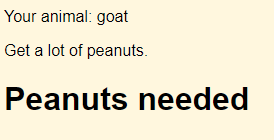Ray

Wait, that's not it! What's going on?

I'll right-click and check the HTML my program made...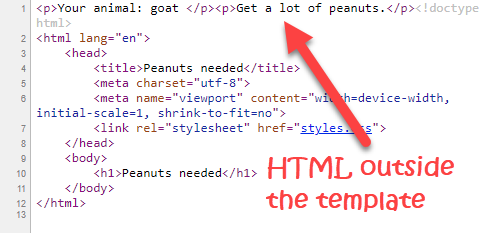Oh, man! I want the animal and peanuts HTML inside the `body`, like this: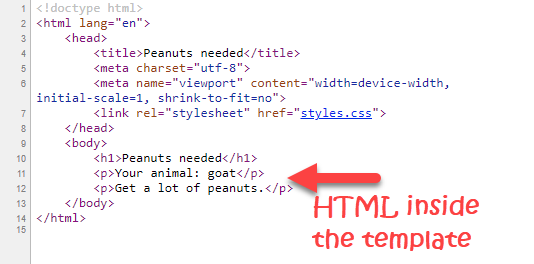Reflect

Here's Ray's code:

1. <?php
2. // Get input.
3. \$animal = \$_GET['animal'];
4. print "<p>Your animal: \$animal </p>";
5. // Print right message.
6. if (\$animal == 'squirrel') {
7.     print '<p>Get a few peanuts.</p>';
8. }
9. elseif (\$animal == 'goat') {
10.     print '<p>Get a lot of peanuts.</p>';
11. }
12. elseif (\$animal == 'elephant') {
13.     print '<p>Get lots and lots and lots of peanuts!</p>';
14. }
15. else {
16.     print "<p>I don't know how many peanuts you'll need.</p>";
17. }
18. ?><!doctype html>
19. <html lang="en">
21.         <title>Peanuts needed</title>
22.         <meta charset="utf-8">
23.         <meta name="viewport" content="width=device-width, initial-scale=1, shrink-to-fit=no">
24.         <link rel="stylesheet" href="styles.css">
26.     <body>
27.         <h1>Peanuts needed</h1>
28.     </body>
29. </html>

What should Ray do to fix the code?

If you were logged in as a student, the lesson would pause here, and you'd be asked to type in a response. If you want to try that out, ask for an account on this site.Ray

What am I going to do?

Well, like Kieran says, when in doubt, find an example.

Indeed!

He's right. I do say that.Ray

Let's see... here's some code from the last lesson:

1. <?php
2. \$dogs = \$_GET['dogs'];
3. // Compute message, and CSS class.
4. \$message = '';
5. \$class = '';
6. if (\$dogs < 1) {
7.     \$message = "You don't have doggos! Boo! Get doggos!";
8.     \$class = 'argh';
9. }
10. ...
11. <!DOCTYPE html>
12. <html lang="en">
13.     <body>
14.         <h1>Dogs</h1>
15.         <p>Do you have enough dogs?</p>
16.         <p>Number of dogs: <?= \$dogs ?></p>
17.         <p class="<?= \$class ?>"><?= \$message ?></p>
18.     ...

Oh, I see it! I print stuff right away:

• <?php
• // Get input.
• \$animal = \$_GET['animal'];
• print "<p>Your animal: \$animal </p>";
• ...
• <!DOCTYPE html>
• <html lang="en">
•     <body>

But the example doesn't. It prints a variable later:

• <?php
• \$dogs = \$_GET['dogs'];
• ...
• ?><!DOCTYPE html>
•     ...
•     <body>
•         <h1>Dogs</h1>
•         <p>Do you have enough dogs?</p>
•         <p>Number of dogs: <?= \$dogs ?></p>

The same in the `if`. I print it immediately:

• if (\$animal == 'squirrel') {
•     print '<p>Get a few peanuts.</p>';
• }
• ...
• <!DOCTYPE html>
• <html lang="en">
•     <body>

The example puts the output it wants into variables, and prints them later:

• \$message = '';
• \$class = '';
• if (\$dogs < 1) {
•     \$message = "You don't have doggos! Boo! Get doggos!";
•     \$class = 'argh';
• }
• ...
• <!DOCTYPE html>
• <html lang="en">
•     <body>
•         ...
•         <p class="<?= \$class ?>"><?= \$message ?></p>
•     ...

Get the order right

You need to output the HTML in the right order, so you make a valid HTML page. Often. that means:

• The top of your code puts the output you want into variables.
• The bottom of your code outputs the variables.Ray

OK, I think I've got it now. I'll use variables.

1. <?php
2. // Set up var to track results.
3. \$peanutsNeeded = '';
4. // Get input.
5. \$animal = \$_GET['animal'];
6. // Adjust peanuts needed.
7. if (\$animal == 'squirrel') {
8.     \$peanutsNeeded = 'Get a few peanuts.';
9. }
10. elseif (\$animal == 'goat') {
11.     \$peanutsNeeded = 'Get a lot of peanuts.';
12. }
13. elseif (\$animal == 'elephant') {
14.     \$peanutsNeeded = 'Get lots and lots and lots of peanuts!';
15. }
16. else {
17.     \$peanutsNeeded = "I don't know how many peanuts you'll need.";
18. }
19. ?><!doctype html>
20. <html lang="en">
22.         <title>Peanuts needed</title>
23.         <meta charset="utf-8">
24.         <meta name="viewport" content="width=device-width, initial-scale=1, shrink-to-fit=no">
25.         <link rel="stylesheet" href="styles.css">
27.     <body>
28.         <h1>Peanuts needed</h1>
29.         <p>Your animal: <?= \$animal ?></p>
30.         <p><?= \$peanutsNeeded ?></p>
31.     </body>
32. </html>Ray

Weehoo! I rock!

When you think about it, you're writing code (in PHP), that writes code (in HTML). Kinda mind blowing.

You have to write your PHP so that it writes the correct HTML. Using variables helps you do that.